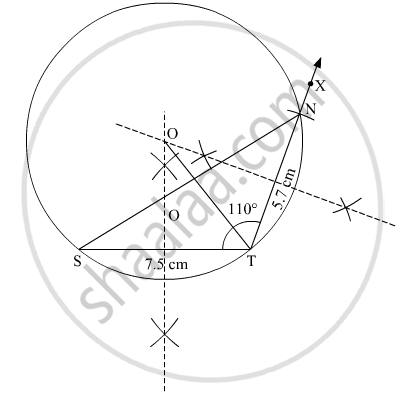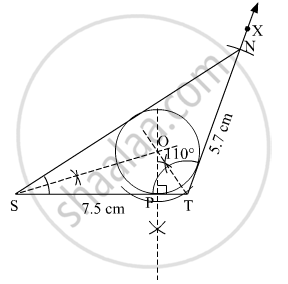Advertisement Remove all ads

# Construct δ Nts Where Nt = 5.7 Cm, Ts = 7.5 Cm and ∠ Nts = 110 ° and Draw Incircle and Circumcircle of It. - Geometry

Diagram

Construct Δ NTS where NT = 5.7 cm, TS = 7.5 cm and
∠ NTS = 110 ° and draw incircle  and circumcircle of it.

Advertisement Remove all ads

#### Solution

Steps of construction:
1. Draw a line ST = 7.5 cm.
2. With T as centre draw an angle ∠ STX = 110°.
3. With T as centre and 5.7 cm as radius, cut an arc on XT and name the point of intersection as N.
4. Join SN. Δ NTS is thus obtained.
5. Draw the perpendicular bisectors of the lines ST and NT. Let these perpendicular bisectors meet at point O.
4. With O as centre and OT as radius, construct a circle touching all the vertices of the Δ NTS.
This circle is thus the required circumcircle.Steps of construction:
1. Draw a line ST = 7.5 cm.
2. With T as centre draw an angle ∠ STX = 110°.
3. With T as centre and 5.7 cm as radius, cut an arc on XT and name the point of intersection as N.
4. Join SN. Δ NTS is thus obtained.
5. Draw the angle bisectors of angle S and T and let them meet at point O.
6. Draw the perpendicular from point O to the line ST. Join OP.
7. With O as centre and OP as radius, draw a circle touching all the sides of the triangle.
This is the required incircle.Concept: Construction of the Incircle of a Triangle.
Is there an error in this question or solution?
Advertisement Remove all ads

#### APPEARS IN

Advertisement Remove all ads
Advertisement Remove all ads
Share
Notifications

View all notifications

Forgot password?